• Call Now

1800-102-2727•

DOT PRODUCT OF TWO VECTORS

We can explain the definition of dot product in two different aspects - in the algebraic sense and the geometric sense. In the algebraic sense, we define a dot product as the sum of the products of the corresponding entries of two given sequences of numbers. In the geometric sense, we define the dot product as the product of the Euclidean magnitudes of the two vectors and the cosine of the angle between them.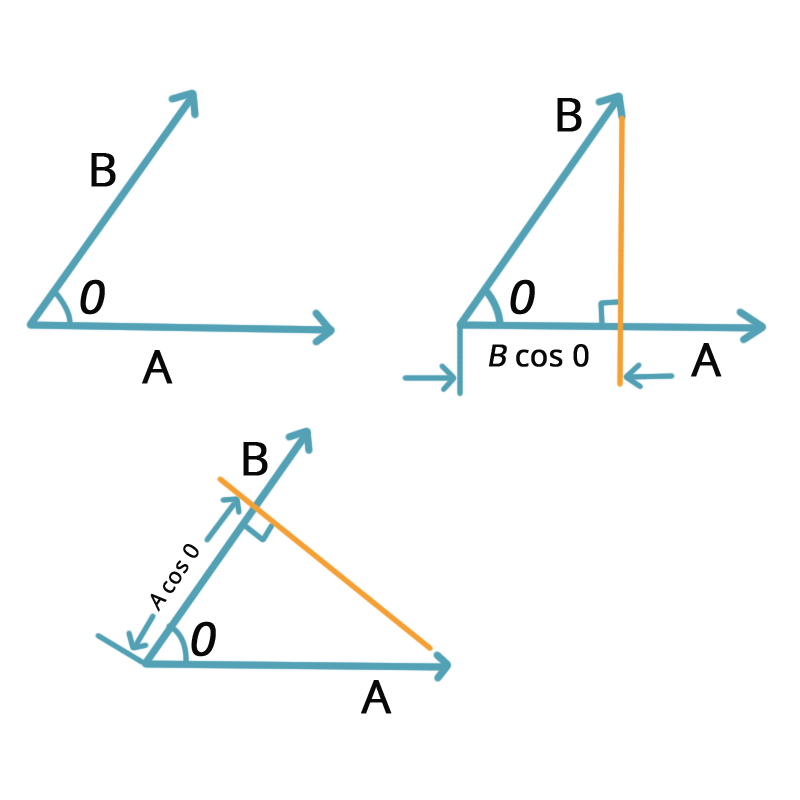But, in a broader sense, we can define the dot product of two vectors as the product of the magnitude of the two vectors and the cos of the angle between them.

The concept of dot products is best understood by the illustration shown below:

o   A, B and cos θ are scalars. The dot product of A and B will also be a scalar quantity.

• B is the product of the magnitude of A and the component of B along with A. Alternatively, it is the product of the magnitude of B and the component of A along B.
• Further, λ A. (λ B) = λ (A.B) where λ is a real number.

SOME CHARACTERISTICS OF A DOT PRODUCT

• The dot product of a. b is a real number
• If a and b are two non-zero vectors, then a. b will be 0, provided a and b are perpendicular to each other.

a. b = 0  a  b

• If the angle θ = 0, then we can say that a. b = |a | |b |.
• If θ = π, then a. b = – |a | |b |.

### ILLUSTRATION OF DOT PRODUCTS

Work (W): The dot product of the force (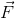) and displacement (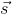) gives Work (W). You would have already studied that work is a scalar quantity that has only magnitude and no direction.

W =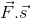= Fs cosθ

Power (P): The dot product of the force () and velocity (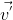) gives Power (P). You would have already studied that power is a scalar quantity that has only magnitude and no direction.

P =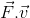### CHARACTERISTICS OF DOT PRODUCT

The two major characteristics of the dot product of two vectors are listed below:

(i) Commutative

The dot product between two vectors follows the property of commutation. To explain this in simple terms, if  aand b  are two vectors, then as per the commutation property we can say that: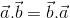This property states that even if the order of the vectors is changed, the final result will not get affected.

(ii) Distributive

The dot product of a vector with the sum of many other vectors is equal to the sum of the dot products of the vector taken with other vectors separately.

Mathematically, it can be expressed as: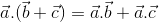Talk to our expert
Resend OTP Timer =
By submitting up, I agree to receive all the Whatsapp communication on my registered number and Aakash terms and conditions and privacy policy